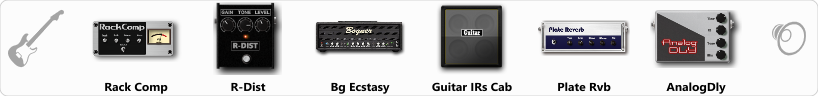# Toska Illumo

Discussion in 'ToneLib-GFX presets' started by Hamilton Ferraz, Sep 10, 2019.

1. Toska Illumo

Preset name: Illumo

Preset based on the tone of the song Illumo of the band Toska.

Effects chain:Effect: "Rack Comp" (Dynamics / Filter), active - "yes"
"Threshold (dB)" = 0
"Ratio" = 10
"Attack" = Fast
"Release (ms)" = 994
"Knee" = 25
"Level (dB)" = 0

Effect: "R-Dist" (Overdrive / Distortion), active - "yes"
"Drive" = 31
"Tone" = 48
"Level" = 50

Effect: "Bg Ecstasy" (Amp simulators), active - "yes"
"Gain" = 38
"Bass" = 38
"Middle" = 74
"Treble" = 66
"Presence" = 38
"Master" = 50
"Output" = 50
"Level (dB)" = 0

Effect: "Guitar IRs Cab" (Cabinets), active - "yes"
"Model" = Bogner Ecstasy (4x12")
"Mic Position" = Center
"Mic Distance" = Near
"Low Cut (Hz)" = 123
"Hi Cut (kHz)" = 20.0
"Mix" = 100
"Level (dB)" = 0

Effect: "Plate Rvb" (Reverb), active - "yes"
"Time" = 4.3
"PreDelay" = 30
"LoDamp" = 17
"HiDamp" = 25
"Mix" = 42

Effect: "AnalogDly" (Delay), active - "yes"
"Time" = 290
"Feedback" = 34
"Tone" = 0
"Mix" = 49

Note: You will need to download and install the ToneLib-GFX software to use the preset.

File size:
560 bytes
Views:
3,102